Next: Model Independent Results Up: Effective Actions in 4D Previous: Tree-level Couplings

## Loop Corrections

We have seen there is good understanding of some of the tree-level couplings of 4D string models. Also non-renormalization theorems guarantee that the superpotential computed at tree-level is exact at all orders in string perturbation theory. This powerful result depends crucially on the fact that the superpotential is a holomorphic function of the fields, so if by the Peccei-Quinn symmetry cannot depend on the imaginary part of the dilaton field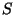, then it cannot depend neither on the real part of. This fact cannot be used for the Kähler potential, which in general will be corrected order by order in string perturbation theory. This is then the least known part of any string theory effective action. On the other hand the gauge kinetic function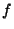is also holomorhic and we know it exactly at the tree-level (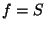). Since this function determines the gauge coupling itself, it is very interesting to consider the loop corrections to.

During the past several years, explicit one-loop corrections tohave been computed, especially for some orbifold models. First it was shown that string loop diagrams reproduce the standard running of the gauge couplings in field theory, as expected. More interesting though, was to find the finite corrections given by threshold effects which include heavy string modes running in the loop. These corrections will be functions of the moduli fields, such as the geometric moduli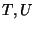but also other moduli such as continuous Wilson lines of orbifold models.

For factorized orbifold models, the explicit dependence of the one-loop corrections on the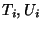moduli fields takes the form :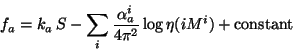(29)

where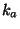are the Kac-Moody levels of the corresponding gauge groups, The coefficients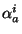are group theoretical quantities depending on the Casimir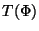of the representation of the matter fields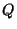and on the Casimir of the adjoint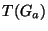as well as on the modular weights of the fields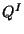. (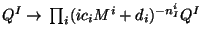.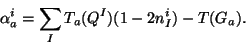(30)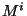refers to the set of both moduli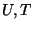for each of the three tori and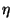is the Dedekind function:(31)

Notice that although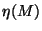transforms in a simple way under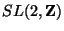transformations, the functionis not invariant underduality. This is as well because the quantitity we need to be invariant is notbut the physical gauge coupling which depends not only onbut also on the Kähler potential at tree-level. Notice the singularity structure of. Using arguments about singularities and invariance of the full gauge coupling, it is possible to extract the expression of the functionin more complicated cases such as Calabi-Yau compactifications, for which the string computation is not possible and the duality grup is not as simple as. Also. extra modifications to equation (29) have been computed recently, including its dependence on continuous Wilson lines .

The calculation of the string one-loop corrections to the gauge couplings is better compared to the Lagrangian for the dilaton in a linear multiplet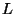. After performing the duality transformation it was found that those are not only corrections to the functionbut also to the Kähler potential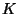of the form (for factorized orbifolds):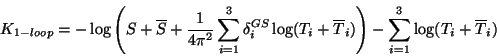(32)

Where the coefficients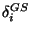are related to a Green-Schwarz counterterm, cancelling duality anomalies, and have been explicitly computed for different orbifolds. This expression forhas been verified by other explicit one-loop calculations, to the first order in a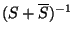expansion .

The knowledge of loop corrections tois not only important for studying questions of gauge coupling unification and supersymmetry breaking by condensation of hidden sector gauge fermions. It is also important because there is also a non-renormalization theorem forstaying that there are no further corrections to f beyond one-loop . This is again as in standard supersymmetric theories . The only thing to keep in mind is that forit is important to state clearly that we are working with the `Wilson' effective action rather than the 1PI effective action. In this case the gauge kinetic function is holomorphic and does not get renormalized beyond one loop . On the other hand, the 1PI gauge coupling is not holomorphic and does get corrections from higher loops but, since it gives the physical coupling, it is invariant under duality symmetries.Next: Model Independent Results Up: Effective Actions in 4D Previous: Tree-level Couplings
root 2001-01-22